# JP4063166B2 - Electric motor control device - Google Patents

## Info

Publication number
JP4063166B2
JP4063166B2 JP2003271330A JP2003271330A JP4063166B2 JP 4063166 B2 JP4063166 B2 JP 4063166B2 JP 2003271330 A JP2003271330 A JP 2003271330A JP 2003271330 A JP2003271330 A JP 2003271330A JP 4063166 B2 JP4063166 B2 JP 4063166B2
Authority
JP
Japan
Prior art keywords
phase angle
feature quantity
axis
current
means
Prior art date
Legal status (The legal status is an assumption and is not a legal conclusion. Google has not performed a legal analysis and makes no representation as to the accuracy of the status listed.)
Expired - Fee Related
Application number
JP2003271330A
Other languages
Japanese (ja)
Other versions
JP2004080986A (en
Inventor

Original Assignee

Priority date (The priority date is an assumption and is not a legal conclusion. Google has not performed a legal analysis and makes no representation as to the accuracy of the date listed.)
Filing date
Publication date
Priority to JP2002222623 priority Critical
Application filed by 日産自動車株式会社 filed Critical 日産自動車株式会社
Priority to JP2003271330A priority patent/JP4063166B2/en
Publication of JP2004080986A publication Critical patent/JP2004080986A/en
Application granted granted Critical
Publication of JP4063166B2 publication Critical patent/JP4063166B2/en
Expired - Fee Related legal-status Critical Current
Anticipated expiration legal-status Critical

• 230000001276 controlling effects Effects 0.000 claims description 3
• 238000000034 methods Methods 0.000 description 6
• 230000000694 effects Effects 0.000 description 1

## Images

•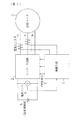•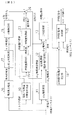•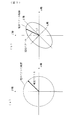•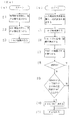•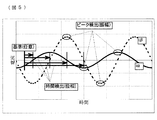••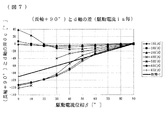•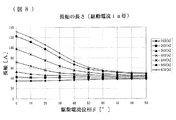•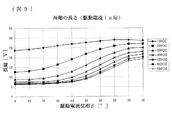•••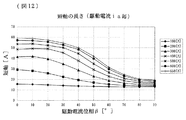••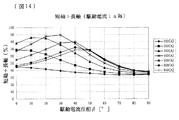•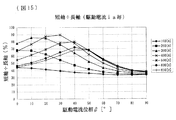•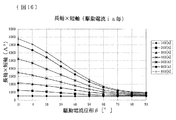•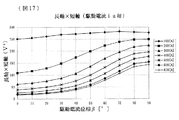••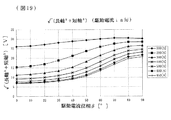•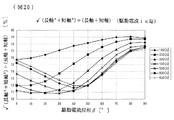••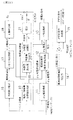••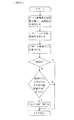••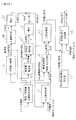•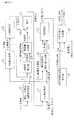•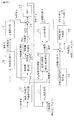•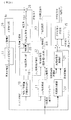•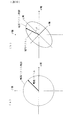••••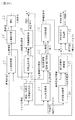••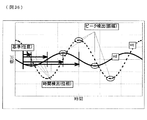•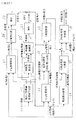•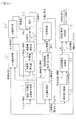•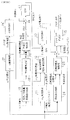## Classifications

• HELECTRICITY
• H02GENERATION; CONVERSION OR DISTRIBUTION OF ELECTRIC POWER
• H02PCONTROL OR REGULATION OF ELECTRIC MOTORS, ELECTRIC GENERATORS OR DYNAMO-ELECTRIC CONVERTERS; CONTROLLING TRANSFORMERS, REACTORS OR CHOKE COILS
• H02P6/00Arrangements for controlling synchronous motors or other dynamo-electric motors using electronic commutation dependent on the rotor position; Electronic commutators therefor
• H02P6/14Electronic commutators
• H02P6/16Circuit arrangements for detecting position
• H02P6/18Circuit arrangements for detecting position without separate position detecting elements
• H02P6/185Circuit arrangements for detecting position without separate position detecting elements using inductance sensing, e.g. pulse excitation
• HELECTRICITY
• H02GENERATION; CONVERSION OR DISTRIBUTION OF ELECTRIC POWER
• H02PCONTROL OR REGULATION OF ELECTRIC MOTORS, ELECTRIC GENERATORS OR DYNAMO-ELECTRIC CONVERTERS; CONTROLLING TRANSFORMERS, REACTORS OR CHOKE COILS
• H02P21/00Arrangements or methods for the control of electric machines by vector control, e.g. by control of field orientation
• H02P21/14Estimation or adaptation of machine parameters, e.g. flux, current or voltage
• H02P21/18Estimation of position or speed
• HELECTRICITY
• H02GENERATION; CONVERSION OR DISTRIBUTION OF ELECTRIC POWER
• H02PCONTROL OR REGULATION OF ELECTRIC MOTORS, ELECTRIC GENERATORS OR DYNAMO-ELECTRIC CONVERTERS; CONTROLLING TRANSFORMERS, REACTORS OR CHOKE COILS
• H02P2207/00Indexing scheme relating to controlling arrangements characterised by the type of motor
• H02P2207/05Synchronous machines, e.g. with permanent magnets or DC excitation

## Description

The present invention relates to a motor control device, for example, a three-phase synchronous motor (internal permanent motor) having a permanent magnet in a rotor.
Magnet Motor: Sensorless control technology in IPM motor).

In order to drive the electric motor with an inverter and control it as a speed control system, it is necessary to detect the magnetic pole position (phase) of the rotor. As a method for detecting the position of the rotor without a sensor, for example, there is a method described in Non-Patent Document 1 below. In this document, the phase is detected by superimposing a high-frequency current on the drive current and detecting the long axis of the high-frequency current vector locus.

"Evaluation of estimation accuracy in position sensorless field detection method of PM motor using current vector locus" National Institute of Electrical Engineers of Japan, No. 683, 1994

The above method has the following problems. That is, the motor has (1) the characteristic that the q-axis inductance decreases as the q-axis current increases and the high-frequency current vector locus approaches a perfect circle due to saturation, and (2) the position where the magnetic flux is most likely to pass due to saturation. There is a characteristic that a position that is difficult to move moves with the current phase. Due to the characteristic of (2) above, the q-axis current increases as a delay due to an error or a delay in the current instruction of the drive current → the detection phase is delayed → When the q-axis current increases into a loop of →..., the elliptic current becomes a perfect circle due to the characteristics of (1), and the correlation of the characteristics of (2) is close to 1, and the major axis of the ellipse is not the d-axis. When the phase is indicated, the control is stepped out, and there is a problem that the sensorless operation cannot be performed at a high load.

The present invention has been made to solve the above-described problems, and an object of the present invention is to provide an electric motor control device capable of sensorless operation even at high loads.

In order to achieve the above object, the present invention is configured as described in the claims. That is, in claim 1, a target value of a feature value based on at least one of a major axis length and a minor axis length of a current vector locus is calculated according to a target torque value, and a drive current for driving an electric motor is calculated as: Superimposing a superimposed current having a frequency different from that of the drive current, detecting a feature quantity actual value based on at least one of a major axis length and a minor axis length of a current vector locus of the superimposed current, and the feature quantity target value The phase angle of the electric motor is detected based on the actual feature value. In other words, by feeding back a feature value obtained from the magnitude of the superimposed current, control is performed to operate the detection phase (when the feature value is larger than the target value, the detection phase is advanced, and when it is smaller, the detection phase is delayed).
As the feature quantity based on at least one of the major axis length and minor axis length of the current vector locus, for example, the major axis length a, minor axis length b, a + b, a × b, √ (a 2 + b 2 ), b / a, √ (a 2 + b 2 ) ÷ (a + b), and the like can be used.

By feeding back a feature value based on at least one of the major axis length and minor axis length of the current vector locus and using it for phase angle detection, the phase angle (rotor magnetic pole position) is sensorless even in a high load region where magnetic saturation occurs. This makes it possible to detect this.

FIG. 1 is a block diagram showing the overall configuration of an embodiment of the present invention.
In FIG. 1, a control means 1 (details will be described later) receives signals from a current sensor 4 and a voltage sensor 6, calculates a PWM (Pulse Width Modulation) command 7, and sends it to the inverter circuit 2. The inverter circuit 2 converts the DC power of the power supply unit 5 into three-phase power corresponding to the PWM command 7 and drives the IPM motor 3 with the power. The current sensor 4 detects a drive current of two phases (for example, U phase and V phase) of the three-phase power sent from the inverter circuit 2 to the IPM motor 3. The voltage sensor 6 detects the output voltage of the power supply unit 5 (input voltage of the inverter 2). The detection values of the current sensor 4 and the voltage sensor 6 are sent to the control means 1 and used for calculation of the PWM command 7. Since the three-phase current has a relationship of U + V + W = 0, if any two phases are detected, the remaining one-phase current can be obtained by calculation.

FIG. 2 is a block diagram of the first embodiment showing details of the control means 1 of FIG.
In FIG. 2, the current / feature quantity target value creation unit 10 generates a d-axis current target value, a q-axis current target value, and a feature quantity target value based on a torque target value (for example, an accelerator pedal operation amount) given from the outside. Create The feature amount is a value based on at least one of the major axis length and the minor axis length of the current vector locus. For example, the major axis length a, the minor axis length b, a + b, a × b, √ (A 2 + b 2 ), a / b, √ (a 2 + b 2 ) / (a + b) (details will be described later).

The d-q axis conversion unit 11 calculates the d-axis current and the q-axis current from the three-phase current and the phase angle θ (detailed later) given from the current sensor 4 of FIG.
The current control unit 12 (current-voltage conversion) performs, for example, PI control (PI control is a known proportional / integral control), and the above-described d-axis current target value and d-axis current, q-axis current target value and q-axis A d-axis voltage command and a q-axis voltage command for controlling to match the current are calculated.
The current control may include non-interference control, or may include a low-pass filter for removing a high-frequency component of the three-phase current.

The three-phase converter 13 calculates and outputs a three-phase voltage command for three phases based on the input two-phase d-axis voltage command, q-axis voltage command, and phase angle θ.
The high-frequency rotational voltage generator 14 (superimposing means for superimposing a superposed current having a frequency higher than the drive current) generates a high-frequency rotational voltage and sends it to the PWM command generator 15. Here, the rotational voltage is a three-phase balanced voltage, and as shown in FIG. 3A, the voltage vector locus becomes a perfect circle when exchanged with the α-β coordinate system which is a two-phase AC coordinate system. State. Since this rotation voltage is a high-frequency voltage that is not synchronized with the IPM motor 3, the IPM motor 3 is not rotated by this rotation voltage.
When there is a difference in inductance between the d-axis and the q-axis as in the IPM motor, the current vector locus becomes an ellipse that swells in the d-axis direction as shown in FIG.

The PWM command creation unit 15 generates a voltage obtained by superimposing the rotation voltage from the high-frequency rotation voltage generation unit 14 and the three-phase voltage command from the three-phase conversion unit 13 and the direct-current voltage supplied from the voltage sensor 6 in FIG. Input and create PWM command 7. The inverter circuit 2 in FIG. 1 is controlled by the PWM command 7 and the IPM motor 3 is driven by the three-phase power output from the inverter circuit 2.

On the other hand, the frequency separation unit 16 (separating means for separating the superimposed current) generally separates and outputs a high-frequency current from the three-phase current input from the current sensor 4 using a frequency filter, and outputs the high-frequency current. The data is sent to the conversion unit 17.
The three-phase to two-phase converter 17 converts the three-phase current of the high frequency component input from the frequency separator 16 into a two-phase current in the α-β coordinate system shown in FIG. The current in the coordinate system is sent to the d-axis detector 18.

The d-axis detector 18 (d-axis phase angle detector) is shown in FIG. 5 for the α-axis component iα and β-axis component iβ of the current vector converted into the α-β coordinate system by the three-phase / two-phase converter 17. As shown, the amplitudes Iα and Iβ are obtained by detecting the peak values, and the superimposed current phases φiα and φiβ are obtained by detecting the time of zero crossing. Then, an angle θ from the α axis to the elliptical long axis (d axis) in the α-β coordinate system shown in FIG.

The angle θ formed by the α axis and the d axis is determined in the range of ± 90 ° by the equation (1).
In order to extend this angle θ to the range of ± 180 °, the assumption that the phase does not change by 90 ° or more in continuous detection is used. That is, the flow shown in FIG. 4 to be described later starts up, for example, every 100 μsec, so that the phase does not normally change by 90 ° or more. Therefore, in the continuous detection, when the angle is changed from 89 ° to −89 °, it is assumed that the angle is changed from 89 ° to 91 ° (= −89 ° + 180 °). Similarly, when the angle is changed from −89 ° to 89 °, it is assumed that the angle is changed from −89 ° to −91 ° (= 89 ° + 180 ° −360 °). Thereby, the angle θ formed by the α axis and the d axis can be detected within a range of ± 180 °.

Note that the initial phase when the first detection is performed cannot be determined because there is no change comparison target. That is, when the calculation result of the first detection is 30 °, it is unknown whether this initial phase is 30 ° or −150 °. Therefore, in the first detection, the range of θ is provisionally set to a range of ± 90 °, and in the above example, 30 °, and the correct θ in the polarity determination by N / S determination described later, that is, θ is ± 180 °. It will be determined in the range.
An angle θ from the α axis to the elliptical long axis (d axis) obtained by the d axis detection unit 18 is defined as a d axis phase angle θ 0 .

The feature quantity calculator 19 (feature quantity actual value detecting means) calculates an actual feature quantity from the α-axis current and the β-axis current (details will be described later).
The feature quantity control unit 20 (correction angle calculation means) calculates the correction angle θ ′ from the actual feature quantity and the feature quantity target value. That is, when the actual feature amount is larger than the feature amount target value, the detection phase is advanced, and when it is smaller, the detection phase is delayed. PI control is used for this feedback control, and the control gain is set experimentally.
The calculation of the correction angle θ ′ by the PI control is performed using the following equation (2) when, for example, the drive current phase angle β increases and the feature value decreases.
As will be described later, when the feature amount is increased as the drive current phase angle β is increased as in the case where the feature amount is √ (a 2 + b 2 ) / (a + b), the equation (2) is used. Becomes (Expression 2 ′).

Note that the feature value target value in the current / feature value target value creation unit 10 includes a method of changing with the current target value, a method of setting a constant value, and the like. Since it is not applied in a small low load region, the control is turned ON / OFF according to the magnitude of the current as described below.
That is, the switch SW1 is turned on only in a high load region where the drive current of the IPM motor 3 (detected by the current sensor 4) is equal to or greater than a predetermined value.
When the switch SW1 is on, the adder 26 (phase angle correction means) uses the d-axis phase angle θ 0 output from the d-axis detection unit 18 and the correction angle θ ′ output from the feature amount control unit 20. Are added, whereby θ 0 is corrected by θ ′, resulting in a phase angle θ. Then, the corrected phase angle θ is sent to the dq axis conversion unit 11 and the three-phase conversion unit 13 and used for the calculation.
Therefore, in the circuit of FIG. 2, in a high load region where the drive current of the IPM motor 3 is equal to or greater than a predetermined value, the phase angle θ corrected based on the feature value is used for the calculation. In the operation state, the d-axis phase angle θ 0 output from the d-axis detector 18 is used as it is.
As described above, if the d-axis phase angle detected by the d-axis detection means 18 is corrected only in a high load region where the drive current of the electric motor is equal to or greater than a predetermined value, correction is necessary. Since the calculation of the correction angle θ ′ in the feature amount calculation unit 19 and the feature amount control unit 20 can be stopped in the low load region where there is no calculation load, the calculation load can be reduced.

FIG. 4 shows a flowchart of processing up to the transition to torque control when there is no magnetic saturation (other than the high load region).
First, in FIG. 4A, in step S1, a provisional d-axis is detected by flowing a high-frequency rotation current corresponding to the high-frequency rotation voltage from the high-frequency rotation voltage generator 14.
In step S2, the true d-axis is detected by performing N / S determination for determining the N pole and the S pole of the magnetic pole. This N / S determination includes (1) a method in which a high frequency voltage is applied as a large voltage that causes saturation due to magnetic flux, and a direction in which the center of the ellipse deviates is detected. A method of comparing the positive and negative magnitudes of the d-axis current by applying wave voltage, (3) Applying positive and negative DC currents only to the d-axis, and comparing the magnitude of the amplitude of the high-frequency current in each case There are methods to do this, and any one of them is used.
As described above, the true d-axis, that is, the d-axis phase angle is detected, and torque control is performed in step S3 using the detected value. This content is as described below the current control unit 12 in FIG.

Next, in FIG.4 (b), in step S4, a high frequency component is isolate | separated from the electric current detected by the current sensor 4 (the said frequency separation part 16). In step S5, the high-frequency current is converted on the α-β axis to obtain the state shown in FIG. 3B (the three-phase / two-phase converter 17).
In step S6, for the α-axis component iα and β-axis component iβ of the current vector converted into the α-β coordinate system, as shown in FIG. 5, amplitudes Iα and Iβ are obtained by detecting peak values, The superimposed current phases φiα and φiβ are obtained by detecting the time of zero crossing.

In step S7, the initial phase θ is provisionally calculated in a range of ± 90 °. Note that the calculated value in this calculation is θ2, and the previous value is θ1.
In steps S8 to S10, the initial phase θ in the ± 90 ° range is expanded to the ± 180 ° range. In order to extend this θ to the range of ± 180 °, the assumption that the phase does not change by 90 ° or more in continuous detection is used. That is, in the case of continuous detection, when the angle is changed from 89 ° to −89 °, it is assumed that the angle is changed from 89 ° to −89 ° + 180 ° = 91 °, and when it is changed from −89 ° to 89 °, It is assumed that the angle has changed from −89 ° to 89 ° + 180 ° = 269 ° = −91 °. Thus, θ can be detected in a range of ± 180 °, but the initial phase is not known when the calculation result is first output. That is, when the first calculation result is 30 °, it is not known whether it is 30 ° or −150 °. Here, the initial phase is provisionally set within a range of ± 90 °.
First, in step S8, it is determined whether or not the current calculation is the first time. In the first time, since it is not possible to determine as described above, the process goes to step S11, and the calculated value is output as it is. If it is not the first time, go to step S9.
In step S9, it is determined whether or not the absolute value of the difference between the previous calculated value θ1 and the current calculated value θ2 is 90 ° or more. If the absolute value is less than 90 °, the process goes to step S11 and the current calculated value θ2 is used as it is. Output. When the absolute value of the difference is 90 ° or more, in step S10, a value obtained by adding 180 ° to the current calculated value θ2 is output.
The above calculation (steps S6 to S11) is performed by the d-axis detector 18.

Next, the correction of the phase angle by the feature amount which is a feature of the present invention will be described.
The correction of the phase angle in the feature quantity calculation unit 19 and the feature quantity control unit 20 is used when magnetic saturation occurs (high load range).
The difference θe between the major axis of the ellipse and the d-axis when magnetic saturation occurs is as shown in FIG. The ellipse means the ellipse (elliptical current) of the current vector locus shown in FIG. In FIG. 6, the vertical axis represents the difference θe between the long axis and the d axis, the horizontal axis β represents the drive current phase angle (phase angle from the q axis), and the drive current i a = √ ( id 2 + i q 2 ). The characteristics are shown. When the drive current value is small, the major axis of the ellipse and the d-axis have some errors, but the major axis does not change to indicate the d-axis (in the range of about 100 A to 300 A in FIG. 6). However, when the drive current value is large, the long axis of the ellipse moves according to the drive current phase angle, and there is no correlation with the d axis, and the correlation with the drive current phase angle is high. In such a state, phase detection cannot be performed. Therefore, when there is magnetic saturation (high load region), another feature amount obtained from the ellipse of the current vector locus is used.

FIG. 7 shows the same content as FIG. 6 with respect to the voltage vector locus (FIG. 30 described later). As shown in FIG. 30B, in the voltage vector locus (voltage ellipse: elliptic voltage), (major axis + 90 °) corresponds to the d axis, so the difference between (major axis + 90 °) and the d axis. θe is displayed.

8, 10, 12, 14, 16, 18, and 20 are diagrams illustrating the relationship between each feature amount and the drive current phase angle β under the same conditions as in FIG. 6. FIG. 10 shows a + b, FIG. 12 shows a short axis length b, FIG. 14 shows b / a, FIG. 16 shows a × b, FIG. 18 shows √ (a 2 + b 2 ), FIG. 20 shows each characteristic of √ (a 2 + b 2 ) ÷ (a + b). 9, FIG. 11, FIG. 15, FIG. 15, FIG. 17, FIG. 19 and FIG. 21 are diagrams showing the relationship between each feature quantity and the drive current phase angle β under the same conditions as in FIG. Is the length a of the major axis in the elliptic voltage, FIG. 11 is a + b, FIG. 13 is the length b of the minor axis, FIG. 15 is b / a, FIG. 17 is a × b, and FIG. 19 is √ (a 2 + b 2 ) FIG. 21 shows each characteristic of √ (a 2 + b 2 ) ÷ (a + b). An embodiment using the above voltage vector locus will be described later with reference to FIG.

In order to control these feature amounts to a predetermined value (a feature amount target value determined by the current / feature amount target value creation unit 10), a feature amount control unit 20 is provided. The feature amount control unit 20 calculates the phase correction angle θ ′ by PI control or the like, and thereby corrects the d-axis phase angle θ 0 . In the characteristics of FIG. 8, FIG. 10, FIG. 12, FIG. 14, FIG. 16, and FIG. 18, the feature quantity has a characteristic that falls to the right according to the drive current phase angle β. When it is larger than the target value, the detection phase is advanced (correction angle θ ′ is set to +), and the drive current phase β is advanced while the drive current phase angle β is kept constant. Similarly, when the feature amount is smaller than the target value, the detection phase is delayed (correction angle θ ′ is −), and the drive current phase angle β is delayed while the drive current phase angle β is kept constant. Put out. At this time, the control gain is experimentally determined. As shown in FIG. 20 (characteristic of √ (a 2 + b 2 ) ÷ (a + b)), when the characteristic amount has a characteristic that rises to the right according to the drive current phase angle β, the relationship between advance / delay Is reversed.

However, the correction by the correction angle θ ′ is performed only in a high load region where magnetic saturation occurs. That is, the switch SW1 is turned on when the drive current of the IPM motor 3 is equal to or greater than a predetermined value, and is turned off when it is small.

As described above, various features such as the length a of the major axis, the length b of the minor axis, a + b, √ (a 2 + b 2 ) and the like can be considered as the characteristic amount obtained from the magnitude of the elliptic current. By using unitless (ratio) feature quantities such as b / a, √ (a 2 + b 2 ) ÷ (a + b), accurate control can be performed.
In addition, when b / a is adopted, it is possible to prevent the elliptic current from becoming a perfect circle. However, in the case of other feature quantities, the feature quantity target value must be set so that the elliptic current does not become a perfect circle. Don't be.
Further, as shown in FIG. 16, in the case of a × b, the current phase angle at the maximum torque is about 30 ° to 50 °, and the slope is different for each current, but is linear and easy to control. This characteristic is the same for a + b and √ (a 2 + b 2 ). However, since the characteristics differ depending on the electric motor, the characteristics are not always good. In the case of a × b, since there is no calculation of √, there is an advantage that the calculation load can be reduced.

The feature amount as described above is calculated by the following formula. That is, if the length of the major axis of the ellipse is a and the length of the minor axis is b, a and b are calculated by the following equation (3).

The current / feature quantity target value creation unit 10 has, as a table for each torque, feature quantities that are previously grasped by experiments as feature quantity target values, and searches and calculates them according to the torque target values in the same manner as the current target values. Since the actual current is different from the current target value, it is desirable to apply a low-pass filter that takes into account the current control response to the feature amount target value. Further, the feature value target value may be calculated from the actual current as a table for each current instead of a table for each torque.

Here, the mathematical formulas used for the calculations described so far are collectively described.

As described above, in the first embodiment, a high load in which magnetic saturation is generated by correcting a phase angle by feeding back a feature amount based on at least one of the major axis length and the minor axis length of a current vector locus. The phase angle can be detected without a sensor even in the region.
Further, it is possible to absorb a delay in phase detection due to a change in the rotational speed.
Further, when “a × b” is used as the feature quantity, similarly to “a + b” and “√ (a 2 + b 2 )”, the maximum torque generation current phase angle is around (about 30 ° to 60 °). There are advantages that the characteristics are good and the amount of calculation is small.

Next, FIG. 22 is a block diagram of the second embodiment showing details of the control means 1 of FIG.
This embodiment shows a configuration in which the phase is detected only by the feature amount control unit 20 ′ without using the d-axis phase angle θ 0 from the d-axis detection unit 18 in a high load region in which magnetic saturation occurs. .
In this case, the feature quantity control unit 20 ′ outputs the angular velocity ω ′ [rad / s], and integrates ω ′ by the integration circuit 21 to calculate the phase angle θ ′ [rad]. That is, the feature amount control unit 20 ′ calculates the angular velocity ω ′ by PI control or the like, and corrects the phase angle. When the feature quantity has a right-down characteristic due to β, if the actual feature quantity is larger than the feature quantity target value, the angular velocity ω ′ is increased, the detection phase is advanced, and the drive current phase angle β remains constant. The effect of advancing the drive current phase angle β is obtained by advancing. When the actual feature amount is smaller than the feature amount target value, the angular velocity ω ′ is lowered, the detection phase is delayed, and the rotational coordinate system is delayed while the drive current phase angle β is kept constant, so that the current phase angle β is delayed. . At this time, the control gain is experimentally determined.
The angular velocity ω ′ is obtained by the following equation (Equation 12). Also, as shown in FIG. 20 (characteristic of (√ (a 2 + b 2 ) ÷ (a + b)), when the characteristic amount has a characteristic that rises to the right according to the drive current phase angle β, the angular velocity ω is It calculates | requires by (Formula 12 ') Formula.

In this case, the relationship between the magnitude of the actual feature value, the feature value target value, and the increase / decrease in ω is opposite to that in the case of having a downward-sloping characteristic.
The switch SW1 ′ is connected to the d-axis detection unit 18 side except in a high load region that causes magnetic saturation, and sends the d-axis phase angle θ 0 from the d-axis detection unit 18 to the subsequent circuit as the phase angle θ. Then, in a high load region where magnetic saturation occurs, switching is made to the integration circuit 21 side, and the value θ ′ calculated by integrating the angular velocity ω is sent to the subsequent circuit as the phase angle θ.
The configuration of FIG. 22 has an advantage that it is not necessary to note that the elliptical current becomes a perfect circle.

Next, FIG. 23 is a block diagram of a third embodiment showing details of the control means 1 of FIG.
This embodiment shows a configuration in which the angular velocity ω is used for the calculation of the d-axis phase angle θ 0 in the d-axis detector 18 ′.
In FIG. 23, the frequency separator 16 receives the d-axis current and the q-axis current converted by the dq-axis converter 11 and separates a high-frequency component (high-frequency dq-axis current) therefrom.
In the d-axis detector 18 ′, the amplitude and phase of the d-axis component id and the q-axis component iq of the high-frequency separated current vector converted on the dq axis are respectively detected by the peak value detection and the zero cross time detection. Ask for. At this time, the angle θ from the d-axis to the ellipse major axis is the same as the equation (1) except that Iα and Iβ are changed to Id and Iq, and φiα and φiβ are changed to φid and φiq. Is multiplied by a constant coefficient (proportional calculation is performed) and output as an angular velocity ω. Then, the integration circuit 22 integrates the ω to generate and output the d-axis phase angle θ 0 .

As described above, the angular velocity ω may be calculated by performing a P (proportional) operation so that the angle θ to the ellipse major axis is 0, but this may be calculated by a PI (proportional integration) operation. good. In addition, the angle θ from the d-axis to the elliptical long axis corresponding to the torque obtained by experiment in advance is held as a table for each torque, and the corresponding angle θ is determined according to the torque target value, thereby improving the phase estimation accuracy. Can be improved.

The feature quantity control unit 20 outputs the correction angle θ ′ as in FIG. 2, and corrects the d-axis phase angle θ 0 by the correction angle θ ′ in the high load region where the switch SW1 is on as in FIG. To the phase angle θ.
When the long axis is detected on the dq axis as shown in FIG. 23, the locus of the high-frequency current vector stops in an elliptical state on the dq axis at a constant rotation, so that there is no detection error due to the rotation. There are advantages.

FIG. 24 is a flowchart of processing up to the transition to torque control when there is no magnetic saturation (other than the high load region), and corresponds to FIG. 4B. The part (a) is the same as FIG.
As shown in FIG. 24, in this flow, after converting the current detected by the current sensor 4 onto the dq axis (dq axis conversion unit 11), the high frequency components are separated, and the current shown in FIG. State. Then, for the d-axis component id and the q-axis component iq of the current vector converted into the dq coordinate system, as shown in FIG. 25, the amplitudes Id and Iq are obtained by detecting the peak value, and the time of zero crossing is obtained. Is detected to obtain superimposed current phases φid and φiq. Subsequent processing is the same as in FIG.

However, when the long axis is detected on the dq axis as in the present embodiment, the equations (Equation 3), (Equation 9), and (Equation 10) in the first and second embodiments described above are used. (Equation 11) and (Equation 11) are represented by the following (Equation 13), (Equation 14), (Equation 15), and (Equation 16), respectively.

In addition, as a method of applying a high-frequency voltage, there is a method of performing on a dq axis (rotating coordinate system) instead of three phases (static coordinate system). That is, in the first to third embodiments, driving is performed by superimposing the high-frequency voltage command output from the high-frequency rotation voltage generator 14 on the three-phase voltage command output from the three-phase converter 13. Although a high-frequency superimposed current is superimposed on the current, a high-frequency voltage command output from the high-frequency rotation voltage generation unit 14 may be superimposed on a dq axis voltage command output from the current control unit 12. The high frequency takes a sufficiently large frequency compared to the rotation frequency (the frequency of the motor drive current), and therefore the influence is small even in the three phases. However, when performed on the dq axes, there is no frequency shift during motor rotation.

Next, FIG. 26 is a block diagram of a fourth embodiment showing details of the control means 1 of FIG. In the present embodiment, as in the third embodiment described above, the d-axis detection unit 18 ′ uses the d-axis component id and the q-axis component iq of the high-frequency separated current vector converted on the dq axis. Respectively, the amplitude and the phase are obtained by detecting the peak value and detecting the time of zero crossing, and are multiplied by a certain coefficient to be output as an angular velocity ω. Then, the integration circuit 22 integrates the ω to generate and output the d-axis phase angle θ 0 .
On the other hand, the feature quantity control unit 20 ′ outputs the angular velocity ω ′ [rad / s] as in the second embodiment, and integrates ω ′ by the integration circuit 21 to obtain the phase angle θ ′ [rad]. calculate.
The switch SW1 ′ is connected to the integration circuit 22 side except in a high load region that causes magnetic saturation, and sends the d-axis phase angle θ 0 from the integration circuit 22 to the subsequent circuit as the phase angle θ. Then, in a high load region where magnetic saturation occurs, switching is made to the integrating circuit 21 side, and the value θ ′ calculated by integrating the angular velocity ω ′ is sent to the subsequent circuit as the phase angle θ.

Next, FIG. 27 is a block diagram of a fifth embodiment showing details of the control means 1 of FIG.
In FIG. 27, the angular velocity ω output from the d-axis detector 18 ′ is sent to the switch SW1 ′ as it is. Further, the feature quantity control unit 20 ′ outputs the angular velocity ω as in FIG. The switch SW1 ′ is connected to the feature amount control unit 20 ′ in a high load region where magnetic saturation occurs, and to the d-axis detection unit 18 ′ in a region other than the high load region. Therefore, the integration circuit 23 integrates the angular velocity ω ′ from the feature amount control unit 20 ′ in the high load region and outputs it as a phase angle θ, and integrates the angular velocity ω from the d-axis detection unit 18 ′ outside the high load region. And output as the phase angle θ.
Note that when θ is switched as shown in FIGS. 22 and 23, the phase jumps when there is an error between the phase calculated by the d-axis detection unit and the phase calculated by the feature amount control unit. This problem is eliminated when ω is switched as in the example.

Next, FIG. 28 is a block diagram of a sixth embodiment showing details of the control means 1 of FIG.
In FIG. 28, the angular velocity ω output from the d-axis detector 18 ′ is sent to the adder 26 as it is. The feature amount control unit 20 ″ outputs the corrected angular velocity ω ″, and in the high load region where the switch SW1 is on, the adder 26 adds the corrected angular velocity ω ″ to the angular velocity ω.
The integrating circuit 23 integrates the output of the adder 26 and outputs it as a phase angle θ. Accordingly, the angular velocity ω from the d-axis detector 18 ′ is integrated and output as the phase angle θ in a region other than the high load region, and the value obtained by correcting the angular velocity ω with the correction angular velocity ω ″ is integrated in the high load region to obtain the phase angle θ. Note that the calculation formula of the corrected angular velocity ω ″ is the same as the formula (Equation 12) and the formula (12 ′), but is clear in the formula (12) and the formula (12 ′). Thus, since the feature amount target value and the feature amount operate so as to coincide with each other, the output of the feature amount control unit 20 ″ becomes the corrected angular velocity ω ″.
In this embodiment, since ω is switched, there is a problem that the phase jumps when there is an error between the phase calculated by the d-axis detection unit and the phase calculated by the feature amount control unit, as in the case of switching θ. Disappear.

Next, FIG. 29 is a block diagram of a seventh embodiment showing details of the control means 1 of FIG.
The first to sixth embodiments described so far superimpose a high frequency current whose voltage vector locus is a perfect circle, and is based on at least one of the length of the major axis and the length of the minor axis of the current vector locus of the high frequency current. In this seventh embodiment, the current vector locus superimposes a perfect circle high frequency current, and the voltage vector locus on the α-β axis of the high frequency current is corrected. The d-axis phase angle is corrected based on at least one of the length of the major axis and the length of the minor axis.

In FIG. 29, the frequency separation unit 24 separates and outputs the high-frequency current from the three-phase current input from the current sensor 4 using a general frequency filter.
The high-frequency current control unit 25 inputs the high-frequency current and the high-frequency current target value, and creates a high-frequency three-phase voltage command by performing PI control or the like so that the high-frequency current vector locus becomes a perfect circle (see FIG. 30a). To do.
As described above, the high-frequency control can be performed not on the three-phase (stationary coordinate system) but on the dq axis (rotational coordinate system).
In this way, when high frequency control is performed on the dq axes and d axis detection or feature value control is performed on the dq axes, there is no frequency shift during motor rotation.

When the high-frequency three-phase voltage command is vectorized, the voltage vector locus becomes an ellipse as shown in FIG. At low load when magnetic saturation does not occur, the major axis direction of the ellipse indicates the q axis (maximum inductance position), and d-axis detection can be performed by subtracting 90 ° from the q-axis phase. That is, the three-phase to two-phase conversion unit 17 inputs the high-frequency three-phase voltage command output from the high-frequency current control unit 25 and outputs an α-β axis voltage as shown in FIG. The d-axis detector 28 calculates and outputs the d-axis phase angle θ 0 by subtracting 90 ° from the q-axis phase as described above.
Note that the phase angle of the major axis of the ellipse (that is, the q axis) can be obtained by an equation in which Iα and Iβ are replaced with Vα and Vβ, and φiα and φiβ are replaced with φvα and φvβ in the equation (1).

In the correction by the feature amount in the high load region, the phase is corrected by keeping the feature amount based on at least one of the major axis length and the minor axis length of the ellipse in FIG. This feature amount is as shown in FIG. 9, FIG. 11, FIG. 13, FIG. 15, FIG. 17, FIG.
In the characteristics shown in FIGS. 9, 11, 13, 17, 19, and 21, since the characteristic amount has a characteristic that rises to the right according to the drive current phase angle β, the actual characteristic amount is characteristic. When the amount is smaller than the target amount, the detection phase is advanced (correction angle θ ′ is +), and the drive current phase angle β is kept constant to advance the rotating coordinate system, thereby advancing the drive current phase β. Similarly, when the feature amount is larger than the target value, the detection phase is delayed (correction angle θ ′ is −), and the drive current phase angle β is delayed while the drive current phase angle β is kept constant, thereby delaying the drive current phase angle β. Put out. At this time, the control gain is experimentally determined. In the case of FIG. 15 (a / b characteristic), the characteristic amount has a right-down characteristic according to the drive current phase angle β. The relationship is reversed from the above.

FIG. 31 is a flowchart of processing up to the transition to torque control when there is no magnetic saturation (other than the high load region), and corresponds to FIG. 4B. The part (a) is the same as FIG.
As shown in FIG. 31, in this flow, the output voltage of the high-frequency current control unit 25 is converted onto the α-β axis to obtain the state shown in FIG. 30B (three-phase two-phase conversion unit 17). Then, for the α-axis component vα and β-axis component vβ of the voltage vector converted into the α-β coordinate system, as shown in FIG. 32, the peak value is detected to obtain the amplitude and phase.
Subsequent processing is the same as in FIG.

When the long axis is detected from the voltage vector locus on the α-β axis as in this embodiment, the equations (Equation 3), (Equation 9), and (Equation 10) in the first embodiment and 2 described above. Expression (Equation 11) becomes the following (Equation 17), (Equation 18), (Equation 19), and (Equation 20).

Next, FIG. 33 is a block diagram of an eighth embodiment showing details of the control means 1 of FIG.
In this embodiment, the d-axis phase angle is corrected based on at least one of the major axis length and minor axis length of the voltage vector locus on the α-β axis of the high-frequency current. The d-axis phase angle θ 0 is calculated, the angular velocity ω ′ detected from the feature quantity is integrated to calculate the phase angle θ ′, and either θ 0 or θ ′ is switched and output. It is. This configuration corresponds to the second embodiment (FIG. 22) using a current vector.

In FIG. 33, the d-axis detector 28 calculates the d-axis phase angle θ 0 based on the α-β-axis voltage vector locus. In addition, the feature quantity control unit 20 ′ calculates an angular velocity ω ′ [rad / s], and integrates it with an integration circuit 21 to calculate a phase angle θ ′ [rad]. And in other than the high load region switches SW1 'is switched to the d-axis detection portion 28 side, and outputs the phase angle theta 0 as the phase angle. On the other hand, in the high load range, the switch SW1 ′ is switched to the integrating circuit 21 side and outputs the phase angle θ ′ as the phase angle.
The angular velocity ω is obtained by the above equation (12).

Next, FIG. 34 is a block diagram of the ninth embodiment showing details of the control means 1 of FIG.
In this embodiment, in the configuration in which the d-axis phase angle is corrected based on at least one of the major axis length and minor axis length of the voltage vector locus on the dq axis of the high-frequency current, This shows a configuration in which the angular velocity ω is used when calculating the d-axis phase angle θ 0 . This configuration corresponds to the third embodiment (FIG. 23) using a current vector.

In the ninth embodiment, the dq axis conversion unit 27 converts the high frequency voltage to the dq axis and outputs the dq axis voltage, and the d axis detection unit 28 'puts the dq axis on the dq axis. The d-axis component vd and the q-axis component vq of the converted voltage vector locus are respectively determined for the amplitude and phase by peak value detection and time detection of 0 cross. At this time, the angle θ from the d-axis to the ellipse major axis is the same as the equation (1) except that Iα and Iβ are changed to Vd and Vq, and φiα and φiβ are changed to φvd and φvq. Is multiplied by a constant coefficient (proportional calculation is performed) and output as an angular velocity ω. Then, the integration circuit 22 integrates the ω to generate and output the d-axis phase angle θ 0 .

As in the third embodiment (FIG. 23), the angular velocity ω may be calculated by a PI (proportional integration) operation, and the elliptical length from the d-axis corresponding to the torque obtained in advance by experiment. By having the angle θ up to the axis as a table for each torque and determining the corresponding angle θ according to the torque target value, the phase estimation accuracy can be improved.
The feature quantity control unit 20 outputs the correction angle θ ′ obtained from the dq-axis voltage, and in the high load region where the switch SW1 is on, the d-axis phase angle θ 0 is set by the correction angle θ ′ as in FIG. The phase angle θ is corrected.

FIG. 35 is a flowchart of processing up to the transition to torque control when there is no magnetic saturation (other than the high load region), and corresponds to FIG. 4B. The part (a) is the same as FIG.
As shown in FIG. 35, in this flow, the output voltage of the high-frequency current control unit 25 is converted onto the dq axes to obtain the state of FIG. Then, for the d-axis component vd and q-axis component vq of the voltage vector converted into the dq coordinate system, as shown in FIG. 36, amplitudes Vd, Vq and phases φvd, φvq are obtained by detecting peak values. .
Subsequent processing is the same as in FIG.

In this embodiment, the long axis is detected on the dq axis as in the third embodiment, so that the locus of the high frequency voltage vector stops in an elliptical state on the dq axis during a fixed rotation. There is an advantage that there is no detection error due to rotation.

However, when the long axis detection is performed on the dq axes as in the present embodiment, (Equation 3), (Equation 9), (Equation 10) in the first embodiment and the second embodiment described above. ) And (Equation 11) are represented by the following (Equation 21), (Equation 22), (Equation 23), and (Equation 24).

Next, FIG. 37 is a block diagram of a tenth embodiment showing details of the control means 1 of FIG. In this embodiment, the d-axis phase angle is corrected based on at least one of the major axis length and the minor axis length of the voltage vector locus on the dq axis of the high-frequency current. When calculating the d-axis phase angle θ 0 , the angular velocity ω is used, and the d-axis phase angle θ ′ obtained from the feature quantity is also calculated using the angular velocity ω ′, and these are switched.

In FIG. 37, the d-axis detector 28 ′ performs peak value detection and zero-cross time detection on the d-axis component vd and the q-axis component vq of the high-frequency separated voltage vector converted on the dq axis, respectively. Then, the amplitude and phase are obtained, multiplied by a certain coefficient, and output as an angular velocity ω. Then, the integration circuit 22 integrates the ω to generate and output the d-axis phase angle θ 0 .
On the other hand, the feature quantity control unit 20 ′ outputs the angular velocity ω ′ [rad / s], and the integration circuit 21 integrates ω ′ to calculate the phase angle θ ′ [rad].
The switch SW1 ′ is connected to the integration circuit 22 side except in a high load region that causes magnetic saturation, and sends the d-axis phase angle θ 0 from the integration circuit 22 to the subsequent circuit as the phase angle θ. Then, in a high load region where magnetic saturation occurs, switching is made to the integrating circuit 21 side, and the value θ ′ calculated by integrating the angular velocity ω ′ is sent to the subsequent circuit as the phase angle θ.

Next, FIG. 38 is a block diagram of an eleventh embodiment showing details of the control means 1 of FIG. In this embodiment, an integrating circuit 23 for integrating the angular velocity ω is connected behind the switch SW1 ′ in the tenth embodiment (FIG. 37).
In FIG. 38, the angular velocity ω output from the d-axis detector 28 ′ is sent to the switch SW1 ′ as it is, and the feature amount controller 20 ′ outputs the angular velocity ω ′ as in FIG. The switch SW1 ′ is connected to the feature amount control unit 20 ′ in a high load region where magnetic saturation occurs, and to the d-axis detection unit 28 ′ in a region other than the high load region. Therefore, the integration circuit 23 integrates the angular velocity ω ′ from the feature amount control unit 20 ′ in the high load region and outputs it as a phase angle θ, and integrates the angular velocity ω from the d-axis detection unit 28 ′ outside the high load region. And output as the phase angle θ.
According to the configuration of FIG. 38, since the switching is performed in the state of the angular velocity ω as in the fifth embodiment (FIG. 27), it is possible to prevent the phase from jumping when the phase angle θ is switched.

FIG. 39 is a block diagram of the twelfth embodiment showing details of the control means 1 of FIG.
In this embodiment, the d-axis phase angle is corrected based on at least one of the major axis length and the minor axis length of the voltage vector locus on the dq axis of the high-frequency current. "Represents a configuration for correction. This configuration corresponds to the sixth embodiment (FIG. 28) applied to the voltage vector method. The calculation formula of the corrected angular velocity ω" is the same as that of the sixth embodiment. Similar to the example, the equation (12) and the equation (12 ′) are used.

In FIG. 39, the angular velocity ω output from the d-axis detector 28 ′ is sent to the adder 26 as it is. The feature amount control unit 20 ″ outputs the corrected angular velocity ω ″, and in the high load region where the switch SW1 is on, the adder 26 adds the corrected angular velocity ω ″ to the angular velocity ω.
The integrating circuit 23 integrates the output ω of the adder 26 and outputs it as a phase angle θ. Therefore, the angular velocity ω from the d-axis detector 28 ′ is integrated and output as the phase angle θ in a region other than the high load region, and the value obtained by correcting the angular velocity ω with the corrected angular velocity ω ″ is integrated in the high load region to obtain the phase angle θ. Since the angular velocity ω is also switched in this embodiment, there is an error between the phase calculated by the d-axis detection unit and the phase calculated by the feature amount control unit as in the case of switching the phase angle θ. , The problem of phase jump is eliminated.

1 is a block diagram showing the overall configuration of an embodiment of the present invention. The block diagram of the 1st Example which shows the detail of the control means 1 of FIG. The figure which shows the voltage vector locus | trajectory of a perfect circle, and the electric current vector locus | trajectory of an ellipse. The flowchart of a process until it transfers to torque control in case there is no magnetic saturation (other than a high load area | region). The figure for demonstrating the detection method of phase (phi) i (alpha) and (phi) i (beta). The figure which shows the difference (theta) e of the major axis of an ellipse in case a magnetic saturation occurs in a current vector locus | trajectory, and d-axis. The figure which shows the difference (theta) e of the major axis of an ellipse in case a magnetic saturation occurs in a voltage vector locus | trajectory, and d-axis. The figure which shows the relationship between the length a of the long axis of the elliptical current which is a feature-value, and electric current phase (beta). The figure which shows the relationship between the length a of the major axis of the elliptical voltage which is a feature-value, and electric current phase (beta). The figure which shows the relationship between the major axis a + short axis b of the elliptical current which is a feature-value, and the electric current phase (beta). The figure which shows the relationship between the major axis a + short axis b of the elliptical voltage which is a feature-value, and the electric current phase (beta). The figure which shows the relationship between the length b of the short axis of the elliptical current which is a feature-value, and electric current phase (beta). The figure which shows the relationship between the short axis length b of the elliptical voltage which is a feature-value, and electric current phase (beta). The figure which shows the relationship between the short axis b / long axis a of the elliptic current which is a feature-value, and current phase (beta). The figure which shows the relationship between the short axis b / long axis a of the elliptical voltage which is a feature-value, and the electric current phase (beta). The figure which shows the relationship between the long axis a x short axis b of the elliptic current which is a feature-value, and electric current phase (beta). The figure which shows the relationship between the major axis axshort axis b of the elliptical voltage which is a feature-value, and current phase (beta). Shows the major axis a and minor axis b of the ellipse current which is a feature quantity, √ and the relationship between the current phase β (a 2 + b 2) . Shows the major axis a and minor axis b of the ellipse voltage which is a feature quantity, √ and the relationship between the current phase β (a 2 + b 2) . Shows the major axis a and minor axis b of the ellipse current which is a feature quantity, √ and (a 2 + b 2) ÷ (a + b) the relationship between the current phase beta. Shows the major axis a and minor axis b of the ellipse voltage which is a feature quantity, √ and (a 2 + b 2) ÷ (a + b) the relationship between the current phase beta. The block diagram of the 2nd Example which shows the detail of the control means 1 of FIG. The block diagram of the 3rd Example which shows the detail of the control means 1 of FIG. The flowchart of a process until it transfers to torque control in a 3rd Example when there is no magnetic saturation (other than a high load area | region). The figure for demonstrating the detection method of phase (phi) id and (phi) iq in a 3rd Example. The block diagram of the 4th Example which shows the detail of the control means 1 of FIG. The block diagram of the 5th Example which shows the detail of the control means 1 of FIG. The block diagram of the 6th Example which shows the detail of the control means 1 of FIG. The block diagram of the 7th Example which shows the detail of the control means 1 of FIG. The figure which shows the current vector locus | trajectory of a perfect circle, and the voltage vector locus | trajectory of an ellipse. The flowchart of a process until it transfers to torque control in the case where there is no magnetic saturation (other than a high load area | region) in a 7th Example. The figure for demonstrating the detection method of phase (phi) v (alpha) and (phi) v (beta) in a 7th Example. The block diagram of the 8th Example which shows the detail of the control means 1 of FIG. The block diagram of the 9th Example which shows the detail of the control means 1 of FIG. The flowchart of a process until it transfers to torque control in the 9th Example when there is no magnetic saturation (except for a high load region). The figure for demonstrating the detection method of phase (phi) vd and (phi) vq in a 9th Example. The block diagram of the 10th Example which shows the detail of the control means 1 of FIG. The block diagram of the 11th Example which shows the detail of the control means 1 of FIG. The block diagram of the 12th Example which shows the detail of the control means 1 of FIG.

Explanation of symbols

DESCRIPTION OF SYMBOLS 1 ... Control means 2 ... Inverter circuit 3 ... IPM motor 4 ... Current sensor 5 ... Power supply part 6 ... Voltage sensor 7 ... PWM command 10 ... Current / feature amount target value preparation part 11 ... dq axis conversion part 12 ... Current control Unit 13 ... three-phase conversion unit 14 ... high-frequency rotational voltage generation unit 15 ... PWM command generation unit 16 ... frequency separation unit 17 ... three-phase two-phase conversion unit 18, 18 '... d-axis detection unit 19 ... feature quantity calculation unit 20, 20 ', 20 "... feature quantity control unit 21, 22, 23 ... integration circuit 24 ... frequency separation unit 25 ... high frequency current control unit 26 ... adder 27 ... dq axis conversion unit 28, 28' ... d axis detection unit

## Claims (38)

1. An inverter circuit that is connected to a DC power source, converts power from the DC power source into AC and outputs the AC, and drives the electric motor with the output AC power, and control for controlling the inverter circuit based on a target torque value An electric motor control device comprising:
Superimposing means for superimposing a superimposed current having a frequency different from the driving current on the driving current for driving the electric motor;
A feature amount target value creating means for calculating a target value of a feature amount based on at least one of a major axis length and a minor axis length of a current vector locus of the superimposed current according to the target torque value;
Separating means for separating the superimposed current from the current supplied to the motor;
Feature quantity actual value detection means for detecting a feature quantity actual value based on at least one of a major axis length and a minor axis length of the current vector locus of the separated superimposed current;
Phase angle detection means for detecting the phase angle of the motor based on the feature quantity target value calculated by the feature quantity target value creation means and the feature quantity actual value detected by the feature quantity actual value detection means;
And the control means controls the inverter circuit based on the phase angle detected by the phase angle detection means.
2. The phase angle detection means includes d-axis phase angle detection means for detecting a d-axis phase angle from a current vector locus of the superimposed current,
The d-axis phase angle detected by the d-axis phase angle detection means, the feature quantity target value calculated by the feature quantity target value creation means, and the feature quantity actual value detected by the feature quantity actual value detection means. The motor control device according to claim 1, wherein the phase angle is detected by performing correction based on the equation (1).
3.   The phase angle detection unit calculates an angular velocity based on the feature amount target value calculated by the feature amount target value creation unit and the feature amount actual value detected by the feature amount actual value detection unit, and the calculation The motor control device according to claim 1, wherein the phase angle is detected based on the measured angular velocity.
4. The phase angle detection means includes
D-axis phase angle detection means for detecting a d-axis phase angle from the current vector locus of the separated superimposed current;
Correction angle calculation means for calculating a correction angle based on the feature quantity target value calculated by the feature quantity target value creation means and the feature quantity actual value detected by the feature quantity actual value detection means;
Phase angle correction means for correcting the d-axis phase angle by the correction angle,
The motor control device according to claim 1, wherein the control unit controls the inverter circuit based on the phase angle corrected by the phase angle correction unit.
5. The phase angle detection means includes
First d-axis phase angle detection means for detecting a d-axis phase angle from the current vector locus of the separated superimposed current;
Based on the feature quantity target value calculated by the feature quantity target value creation means and the feature quantity actual value detected by the feature quantity actual value detection means, the angular velocity ω is detected, and the detected angular speed ω is integrated. Second d-axis phase angle calculating means for calculating the d-axis phase angle;
Switching means for switching between the first and second d-axis phase angle detection means and outputting either one of the d-axis phase angles;
The motor control device according to claim 1, wherein the control unit controls the inverter circuit based on a phase angle output from the switching unit.
6. The phase angle detection means includes
D-axis phase angle detection means for detecting an angular velocity from the current vector locus of the separated superimposed current and integrating it to detect a d-axis phase angle;
Correction angle calculation means for calculating a correction angle based on the feature quantity target value calculated by the feature quantity target value creation means and the feature quantity actual value detected by the feature quantity actual value detection means;
Phase angle correction means for correcting the d-axis phase angle by the correction angle,
The motor control device according to claim 1, wherein the control unit controls the inverter circuit based on the phase angle corrected by the phase angle correction unit.
7. The phase angle detection means includes
First d-axis phase angle detecting means for detecting an angular velocity from the current vector locus of the separated superimposed current and integrating it to detect a d-axis phase angle;
Based on the feature quantity target value calculated by the feature quantity target value creation means and the feature quantity actual value detected by the feature quantity actual value detection means, the angular velocity ω is detected, and the detected angular speed ω is integrated. Second d-axis phase angle calculating means for calculating the d-axis phase angle;
Switching means for switching between the first and second d-axis phase angle detection means and outputting either one of the d-axis phase angles;
The motor control device according to claim 1, wherein the control unit controls the inverter circuit based on a phase angle output from the switching unit.
8. The phase angle detection means includes
Angular velocity detection means for detecting angular velocity from the current vector locus of the separated superimposed current;
Correction angular velocity calculation means for calculating a correction angular velocity based on the feature quantity target value calculated by the feature quantity target value creation means and the feature quantity actual value detected by the feature quantity actual value detection means;
Angular velocity correction means for correcting the angular velocity by the corrected angular velocity;
Phase angle calculation means for calculating the phase angle by integrating the output of the angular velocity correction means,
The motor control device according to claim 1, wherein the control unit controls the inverter circuit based on the phase angle calculated by the phase angle calculation unit.
9. The phase angle detection means includes
First angular velocity detection means for detecting an angular velocity from the current vector locus of the separated superimposed current;
Second angular velocity detection means for detecting angular velocity ω based on the feature quantity target value calculated by the feature quantity target value creation means and the feature quantity actual value detected by the feature quantity actual value detection means;
Switching means for switching between the first and second angular velocity detecting means and outputting either one of the angular velocities;
Phase angle calculating means for calculating the phase angle by integrating the output of the switching means,
The motor control device according to claim 1, wherein the control unit controls the inverter circuit based on the phase angle output from the phase angle calculation unit.
10.   The phase angle correction means corrects the d-axis phase angle detected by the d-axis phase angle detection means only in a high load region where the drive current of the electric motor is equal to or greater than a predetermined value. The motor control device according to claim 4 or claim 6.
11.   The switching means outputs the d-axis phase angle detected by the second d-axis phase angle detection means only in a high load region where the drive current of the electric motor is not less than a predetermined value. The motor control device according to claim 5 or 7.
12.   9. The electric motor according to claim 8, wherein the angular velocity correcting unit corrects the angular velocity detected by the angular velocity detecting unit only in a high load region where a driving current of the electric motor is equal to or greater than a predetermined value. Control device.
13.   The said switching means outputs the angular velocity detected by the said 2nd angular velocity detection means only in the high load area | region where the drive current of an electric motor is more than the predetermined value defined beforehand. Electric motor control device.
14.   The product a × b of a major axis length a and a minor axis length b of a current vector locus of a superimposed current is used as the feature quantity target value and the feature quantity actual value. The motor control device according to any one of 13.
15.   The ratio b / a between the minor axis length b and the major axis length a of the current vector locus of the superimposed current is used as the feature quantity target value and the feature quantity actual value. The motor control device according to any one of 13.
16. As the feature quantity target value and the feature quantity actual value, the major axis length a and minor axis length b of the current vector locus of the superimposed current are expressed by the following formula √ (a 2 + b 2 ) ÷ (a + b)
The motor control device according to claim 1, wherein a value obtained by substituting is used.
17.   6. The motor control device according to claim 1, wherein the current vector locus of the superimposed current is a current vector locus on the α-β axis.
18.   10. The motor control according to claim 1, wherein the current vector locus of the superimposed current is a current vector locus on a dq axis. 11. apparatus.
19.   The superimposed current is a high-frequency current with a voltage vector locus of a perfect circle, and the phase angle is determined based on at least one of a major axis length and a minor axis length of the current vector locus of the high-frequency current by superimposing the high-frequency current. 19. The motor control device according to claim 1, wherein the motor control device is detected.
20. An inverter circuit that is connected to a DC power source, converts power from the DC power source into AC and outputs the AC, and drives the electric motor with the output AC power, and control for controlling the inverter circuit based on a target torque value An electric motor control device comprising:
Superimposing means for superimposing a superimposed current having a frequency different from the driving current on the driving current for driving the electric motor;
A feature amount target value creating means for calculating a target value of a feature amount based on at least one of a major axis length and a minor axis length of the voltage vector locus of the superimposed current according to the target torque value;
Separating means for separating the superimposed current from the current supplied to the motor;
Feature quantity actual value detection means for detecting a feature quantity actual value based on at least one of a major axis length and a minor axis length of the voltage vector locus of the separated superimposed current;
Phase angle detection means for detecting the phase angle of the motor based on the feature quantity target value calculated by the feature quantity target value creation means and the feature quantity actual value detected by the feature quantity actual value detection means;
And the control means controls the inverter circuit based on the phase angle detected by the phase angle detection means.
21. The phase angle detection means includes d-axis phase angle detection means for detecting a d-axis phase angle from the voltage vector locus of the superimposed current,
The d-axis phase angle detected by the d-axis phase angle detection means, the feature quantity target value calculated by the feature quantity target value creation means, and the feature quantity actual value detected by the feature quantity actual value detection means. 21. The motor control device according to claim 20, wherein the phase angle is detected by performing correction based on.
22.   The phase angle detection unit calculates an angular velocity based on the feature amount target value calculated by the feature amount target value creation unit and the feature amount actual value detected by the feature amount actual value detection unit, and the calculation 21. The motor control device according to claim 20, wherein the phase angle is detected based on the measured angular velocity.
23. The phase angle detection means includes
D-axis phase angle detection means for detecting a d-axis phase angle from the voltage vector locus of the separated superimposed current;
Correction angle calculation means for calculating a correction angle based on the feature quantity target value calculated by the feature quantity target value creation means and the feature quantity actual value detected by the feature quantity actual value detection means;
Phase angle correction means for correcting the d-axis phase angle by the correction angle,
21. The motor control device according to claim 20, wherein the control unit controls the inverter circuit based on the phase angle corrected by the phase angle correction unit.
24. The phase angle detection means includes
First d-axis phase angle detecting means for detecting a d-axis phase angle from the voltage vector locus of the separated superimposed current;
Based on the feature quantity target value calculated by the feature quantity target value creation means and the feature quantity actual value detected by the feature quantity actual value detection means, the angular velocity ω is detected, and the detected angular speed ω is integrated. Second d-axis phase angle calculating means for calculating the d-axis phase angle;
Switching means for switching between the first and second d-axis phase angle detection means and outputting either one of the d-axis phase angles;
21. The motor control apparatus according to claim 20, wherein the control unit controls the inverter circuit based on a phase angle output from the switching unit.
25. The phase angle detection means includes
D-axis phase angle detection means for detecting an angular velocity from the voltage vector locus of the separated superimposed current and integrating it to detect a d-axis phase angle;
Correction angle calculation means for calculating a correction angle based on the feature quantity target value calculated by the feature quantity target value creation means and the feature quantity actual value detected by the feature quantity actual value detection means;
Phase angle correction means for correcting the d-axis phase angle by the correction angle,
21. The motor control device according to claim 20, wherein the control unit controls the inverter circuit based on the phase angle corrected by the phase angle correction unit.
26. The phase angle detection means includes
First d-axis phase angle detection means for detecting an angular velocity from the voltage vector locus of the separated superimposed current and integrating it to detect a d-axis phase angle;
Based on the feature quantity target value calculated by the feature quantity target value creation means and the feature quantity actual value detected by the feature quantity actual value detection means, the angular velocity ω is detected, and the detected angular speed ω is integrated. Second d-axis phase angle calculating means for calculating the d-axis phase angle;
Switching means for switching between the first and second d-axis phase angle detection means and outputting either one of the d-axis phase angles;
21. The motor control apparatus according to claim 20, wherein the control unit controls the inverter circuit based on a phase angle output from the switching unit.
27. The phase angle detection means includes
Angular velocity detection means for detecting an angular velocity from the voltage vector locus of the separated superimposed current;
Correction angular velocity calculation means for calculating a correction angular velocity based on the feature quantity target value calculated by the feature quantity target value creation means and the feature quantity actual value detected by the feature quantity actual value detection means;
Angular velocity correction means for correcting the angular velocity by the corrected angular velocity;
Phase angle calculation means for calculating the phase angle by integrating the output of the angular velocity correction means,
21. The motor control device according to claim 20, wherein the control unit controls the inverter circuit based on the phase angle calculated by the phase angle calculation unit.
28. The phase angle detection means includes
First angular velocity detection means for detecting an angular velocity from the voltage vector locus of the separated superimposed current;
Second angular velocity detection means for detecting angular velocity ω based on the feature quantity target value calculated by the feature quantity target value creation means and the feature quantity actual value detected by the feature quantity actual value detection means;
Switching means for switching between the first and second angular velocity detecting means and outputting either one of the angular velocities;
Phase angle calculating means for calculating the phase angle by integrating the output of the switching means,
21. The motor control device according to claim 20, wherein the control unit controls the inverter circuit based on the phase angle output from the phase angle calculation unit.
29.   The phase angle correction means corrects the d-axis phase angle detected by the d-axis phase angle detection means only in a high load region where the drive current of the electric motor is equal to or greater than a predetermined value. Item 26. The motor control device according to Item 23 or 25.
30.   The switching means outputs the d-axis phase angle detected by the second d-axis phase angle detection means only in a high load region where the drive current of the electric motor is not less than a predetermined value. 27. A motor control device according to claim 24 or claim 26.
31.   28. The electric motor according to claim 27, wherein the angular velocity correcting means corrects the angular velocity detected by the angular velocity detecting means only in a high load region where a driving current of the electric motor is equal to or greater than a predetermined value. Control device.
32.   The switching means outputs the angular velocity detected by the second angular velocity detecting means only in a high load region where the drive current of the electric motor is equal to or greater than a predetermined value. Electric motor control device.
33.   21. The product a × b of a major axis length a and a minor axis length b of a voltage vector locus of a superimposed current is used as the feature quantity target value and the feature quantity actual value. 32. The motor control device according to any one of 32.
34.   21. The ratio b / a between the short axis length b and the long axis length a of the voltage vector locus of the superimposed current is used as the feature amount target value and the feature amount actual value. 32. The motor control device according to any one of 32.
35. As the feature quantity target value and the feature quantity actual value, the major axis length a and minor axis length b of the voltage vector locus of the superimposed current are expressed by the following formulas √ (a 2 + b 2 ) ÷ (a + b)
33. The motor control apparatus according to claim 20, wherein a value obtained by substituting for is used.
36.   The motor control apparatus according to any one of claims 20 to 24, wherein the voltage vector locus of the superimposed current is a voltage vector locus on the α-β axis.
37.   29. The motor control apparatus according to claim 20, wherein the voltage vector locus of the superimposed current is a voltage vector locus on a dq axis. .
38.   The superposed current is a high-frequency current having a current vector locus of a perfect circle, and this high-frequency current is superposed so that the phase angle is determined based on at least one of the major axis length and the minor axis length of the voltage vector locus of the high-frequency current. The motor control device according to any one of claims 20 to 37, wherein the motor control device is detected.
JP2003271330A 2002-07-31 2003-07-07 Electric motor control device Expired - Fee Related JP4063166B2 (en)

## Priority Applications (2)

Application Number Priority Date Filing Date Title
JP2002222623 2002-07-31
JP2003271330A JP4063166B2 (en) 2002-07-31 2003-07-07 Electric motor control device

## Applications Claiming Priority (2)

Application Number Priority Date Filing Date Title
JP2003271330A JP4063166B2 (en) 2002-07-31 2003-07-07 Electric motor control device
US10/629,896 US7005828B2 (en) 2002-07-31 2003-07-30 Control device for electric motor

## Publications (2)

Publication Number Publication Date
JP2004080986A JP2004080986A (en) 2004-03-11
JP4063166B2 true JP4063166B2 (en) 2008-03-19

# Family

## Family Applications (1)

Application Number Title Priority Date Filing Date
JP2003271330A Expired - Fee Related JP4063166B2 (en) 2002-07-31 2003-07-07 Electric motor control device

## Country Status (2)

US (1) US7005828B2 (en)
JP (1) JP4063166B2 (en)

## Families Citing this family (30)

* Cited by examiner, † Cited by third party
Publication number Priority date Publication date Assignee Title
DE60332058D1 (en) * 2003-08-28 2010-05-20 Mitsubishi Electric Corp Control of a turning machine
GB2410847A (en) * 2004-02-05 2005-08-10 Dyson Ltd Control of motor winding energisation according to rotor angle
US7960938B2 (en) 2004-12-21 2011-06-14 Rockwell Automation Technologies, Inc. Method and apparatus for determining motor rotation status
JP4811145B2 (en) * 2005-06-14 2011-11-09 日産自動車株式会社 Multi-phase motor rotation angle detector
US7667426B2 (en) * 2005-06-17 2010-02-23 Gm Global Technology Operations, Inc. On-line minimum copper loss control of interior permanent-magnet synchronous machine for automotive applications
JP4425193B2 (en) 2005-08-16 2010-03-03 三洋電機株式会社 Motor position sensorless control device
JP4716118B2 (en) 2006-03-29 2011-07-06 株式会社ジェイテクト Motor control device
US7288923B1 (en) * 2006-04-21 2007-10-30 Pratt & Whitney Canada Corp. Voltage-limited electric machine
JP4928855B2 (en) * 2006-07-05 2012-05-09 株式会社東芝 Sensorless control device for synchronous machine
JP4905777B2 (en) * 2006-08-10 2012-03-28 富士電機株式会社 AC / AC direct converter controller
JP4770639B2 (en) * 2006-08-17 2011-09-14 アイシン・エィ・ダブリュ株式会社 Electric motor drive control method and apparatus
US7932692B2 (en) 2006-11-13 2011-04-26 Denso Corporation Control system for rotary electric machine with salient structure
JP4988374B2 (en) * 2007-02-15 2012-08-01 三洋電機株式会社 Motor control device
JP2008220096A (en) 2007-03-06 2008-09-18 Toshiba Corp Sensorless controller of synchronous electric motor
WO2009040965A1 (en) * 2007-09-27 2009-04-02 Mitsubishi Electric Corporation Controller of rotary electric machine
JP5145850B2 (en) * 2007-10-09 2013-02-20 株式会社デンソー Rotating machine control device
JP5402106B2 (en) * 2009-03-10 2014-01-29 日産自動車株式会社 Electric motor control device and electric motor state estimation method
JP5402105B2 (en) * 2009-03-10 2014-01-29 日産自動車株式会社 Electric motor control device and electric motor state estimation method
IT1394426B1 (en) * 2009-06-05 2012-06-15 Reel S R L Unipersonale of an engine control method
DE102010044783A1 (en) * 2010-03-02 2011-09-08 Grob-Werke Gmbh & Co. Kg processing machine
JP5481286B2 (en) * 2010-06-30 2014-04-23 日立オートモティブシステムズ株式会社 Power conversion system and power conversion device
DE102010031430A1 (en) * 2010-07-16 2012-01-19 Robert Bosch Gmbh A method or voltage detection system for determining a correction parameter for a measurement channel and for detecting a terminal voltage of an electric motor
JP5194083B2 (en) * 2010-09-22 2013-05-08 山洋電気株式会社 Method and apparatus for determining deterioration of permanent magnet of electrical equipment
EP2787621A4 (en) * 2011-11-30 2015-09-09 Yaskawa Denki Seisakusho Kk Matrix converter
GB2503039B (en) 2012-06-15 2020-05-27 Danfoss Drives As Method for controlling a synchronous reluctance electric motor
GB2503040B (en) * 2012-06-15 2020-05-06 Danfoss Drives As Variable torque angle for electric motor
EP2940858B1 (en) * 2012-12-28 2019-06-19 Nissan Motor Co., Ltd Motor control device and motor control method
EP2959575A2 (en) * 2013-02-20 2015-12-30 TRW Limited Motor control circuit and method of monitoring a motor
US9571024B1 (en) * 2016-01-05 2017-02-14 Caterpillar Inc. Multiphase motor generator system and control method thereof
WO2018025319A1 (en) * 2016-08-02 2018-02-08 三菱電機株式会社 Control device for rotating electric machine

## Family Cites Families (10)

* Cited by examiner, † Cited by third party
Publication number Priority date Publication date Assignee Title
JPH05199796A (en) * 1992-01-17 1993-08-06 Meidensha Corp Current control system for adjustable speed driving device
US5959430A (en) * 1997-03-07 1999-09-28 Kabushiki Kaisha Toshiba Power conversion system
US6552509B2 (en) * 2000-05-10 2003-04-22 Gti Electroproject B.V. Method and a device for sensorless estimating the relative angular position between the stator and rotor of a three-phase synchronous motor
EP1198059A3 (en) * 2000-10-11 2004-03-17 Matsushita Electric Industrial Co., Ltd. Method and apparatus for position-sensorless motor control
JP3502040B2 (en) * 2000-12-27 2004-03-02 本田技研工業株式会社 Brushless DC motor constant detection device, brushless DC motor control device, and brushless DC motor constant detection program
JP4687846B2 (en) * 2001-03-26 2011-05-25 株式会社安川電機 Magnetic pole position estimation method and control apparatus for synchronous motor
JP3726713B2 (en) * 2001-06-14 2005-12-14 日産自動車株式会社 Electric motor control device
JP2003037990A (en) * 2001-07-24 2003-02-07 Hitachi Ltd Motor control device
JP3801906B2 (en) * 2001-11-07 2006-07-26 株式会社日立カーエンジニアリング Electric vehicle control device and control method
JP3695436B2 (en) * 2002-09-18 2005-09-14 株式会社日立製作所 Position sensorless motor control method and apparatus

## Also Published As

Publication number Publication date
JP2004080986A (en) 2004-03-11
US20040100222A1 (en) 2004-05-27
US7005828B2 (en) 2006-02-28

## Similar Documents

Publication Publication Date Title
US8988027B2 (en) Motor control apparatus and motor control method
JP5324159B2 (en) Motor control device
EP1873901B1 (en) Motor control device
JP3755424B2 (en) AC motor drive control device
US6628099B2 (en) Synchronous motor driving system and sensorless control method for a synchronous motor
US6462491B1 (en) Position sensorless motor control apparatus
JP3627683B2 (en) Motor control device
US6674262B2 (en) Motor control apparatus and motor control method
JP4687846B2 (en) Magnetic pole position estimation method and control apparatus for synchronous motor
DE112007000277B4 (en) Control method and apparatus for an electric motor
US6344725B2 (en) Method and apparatus for controlling a synchronous motor
JP4082444B1 (en) Vector controller for permanent magnet synchronous motor
US6501243B1 (en) Synchronous motor-control apparatus and vehicle using the control apparatus
JP4674516B2 (en) Method for estimating magnetic pole position of synchronous motor
US7808203B2 (en) Motor control device
US8674638B2 (en) Determining initial rotor position of an alternating current motor
JP5069306B2 (en) Control device for rotating electrical machine
US7573227B2 (en) Controller and control method of permanent magnet type rotary motor
JP4067949B2 (en) Motor control device
KR101109067B1 (en) Position sensorless control apparatus of permanent magnet motor
US10158305B2 (en) Inverter controller and motor driving system
JP3982232B2 (en) Sensorless control device and control method for synchronous generator
US7932692B2 (en) Control system for rotary electric machine with salient structure
JP4425193B2 (en) Motor position sensorless control device
US7187155B2 (en) Leakage inductance saturation compensation for a slip control technique of a motor drive

## Legal Events

Date Code Title Description
A621 Written request for application examination

Free format text: JAPANESE INTERMEDIATE CODE: A621

Effective date: 20060529

TRDD Decision of grant or rejection written
A01 Written decision to grant a patent or to grant a registration (utility model)

Free format text: JAPANESE INTERMEDIATE CODE: A01

Effective date: 20071211

A61 First payment of annual fees (during grant procedure)

Free format text: JAPANESE INTERMEDIATE CODE: A61

Effective date: 20071224

R150 Certificate of patent or registration of utility model

Free format text: JAPANESE INTERMEDIATE CODE: R150

FPAY Renewal fee payment (event date is renewal date of database)

Free format text: PAYMENT UNTIL: 20110111

Year of fee payment: 3

FPAY Renewal fee payment (event date is renewal date of database)

Free format text: PAYMENT UNTIL: 20120111

Year of fee payment: 4

FPAY Renewal fee payment (event date is renewal date of database)

Free format text: PAYMENT UNTIL: 20130111

Year of fee payment: 5

FPAY Renewal fee payment (event date is renewal date of database)

Free format text: PAYMENT UNTIL: 20130111

Year of fee payment: 5

FPAY Renewal fee payment (event date is renewal date of database)

Free format text: PAYMENT UNTIL: 20140111

Year of fee payment: 6

LAPS Cancellation because of no payment of annual fees